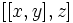# Free product of class two of two elementary abelian groups of prime-square order

View other such prime-parametrized groups

## Definition

Let$p$ be a prime number. This group is defined as the quotient of the free product of two copies of the elementary abelian group of prime-square order with prime$p$, by the third member of its lower central series. In other words, it is the free product in the sense of groups of nilpotency class two of two copies of the elementary abelian subgroup of order$p^2$.

An explicit presentation is given as follows. If we define:$K := \langle a_1, a_2, b_1, b_2 \mid a_1a_2 = a_2a_1, b_1b_2 = b_2b_1, a_1^p = a_2^p = b_1^p = b_2^p = e\rangle$

Then our group is:$G := K/[[K,K],K]$

(this can be written down in a single presentation by writing all the nontrivial commutators$[[x,y],z]$ with$x,y,z \in \{a_1,a_2,b_1,b_2\}$.

## Facts

• The case$p = 2$ give the free product of class two of two Klein four-groups. This group has group ID$(256,8935)$. The semidirect product of this group and a cyclic group of order two that acts by interchanging the$a_i$s and$b_i$s is a group of order$2^9 = 512$. The latter gives an example of the fact that abelian-to-normal replacement fails for prime-sixth order for prime equal to two.
• For all$p$, this group has exactly two abelian subgroups of order$p^6$, both of which are elementary abelian normal subgroups. Thus, for all$p$, this shows that the congruence condition fails for abelian subgroups of prime-sixth order.